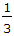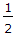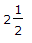# Civil Engineering - Soil Mechanics and Foundation Engineering

### Exercise :: Soil Mechanics and Foundation Engineering - Section 5

31.

The Mohr's straight theory is based on the following fact :

 A. Material fails essentially by shear B. Ultimate strength of the material is determined by the stress in the plane of slip C. Failure criterion is independent of the intermediate principal stress D. All the above.

Explanation:

No answer description available for this question. Let us discuss.

32.

 A. temperature correction B. meniscus correction C. dispersing agent correction D. meniscus and dispersing agent corrections E. temperature, meniscus and dispersing agent corrections.

Explanation:

No answer description available for this question. Let us discuss.

33.

Tergazhi's theory of one dimensional consolidation assumes

 A. soil is homogeneous and fully saturated B. water and soil particles are incompressible C. deformation of the soil, is entirely due to change in volume D. Darcey's law for the velocity of flow of water through soil, is perfectly valid E. all the above.

Explanation:

No answer description available for this question. Let us discuss.

34.

An unsaturated 100 cm3 sample of soil weighs 190 g. If its dried weight is 160 g, water content of the soil, is

 A. 0.188 B. 0.288 C. 0.388 D. 0.488 E. 0.588

Explanation:

No answer description available for this question. Let us discuss.

35.

If the natural moisture content, the liquid limit and plastic limit of a soil sample are stated as 30.5%, 42.5% and 22.5% respectively, the ratio of liquidity index and plastic index, is

 A.B.C. 2 D.